###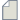Author Topic: Calculate the magnetic moment for PAW calculations  (Read 183 times)

0 Members and 1 Guest are viewing this topic.

####maj7293

• Regular QuantumATK user
•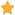• Posts: 5
• Country: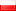• Reputation: 0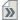##### Calculate the magnetic moment for PAW calculations
« on: August 17, 2023, 17:01 »
Dear all,

I have calculate the Spin orbit calculations of Eu using PAW calculator (SO-GGA) and I need now to calculate the magnetic moment per atom but ATK doesn't have the module for calculating MullikenPopulation. Is there anyone to help me how can I calculate the magnetic moment for this type of calculations?

* Of course if anybody know how can I find the fully relativistic pseudo potential for LCAO calculator, I will be appreciated.

####Anders Blom

• QuantumATK Staff
• Supreme QuantumATK Wizard
•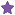• Posts: 5287
• Country:• Reputation: 88##### Re: Calculate the magnetic moment for PAW calculations
« Reply #1 on: August 17, 2023, 20:30 »